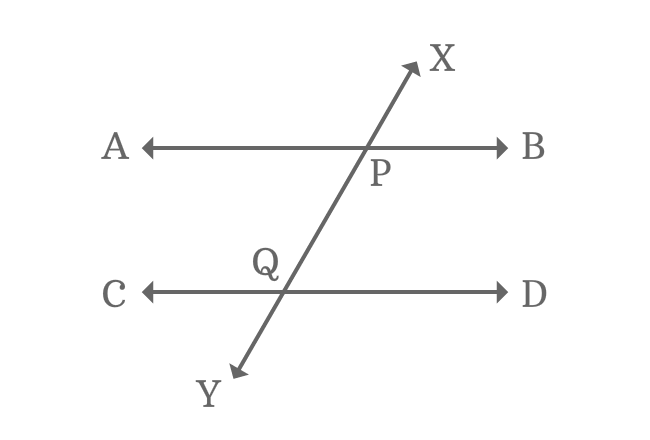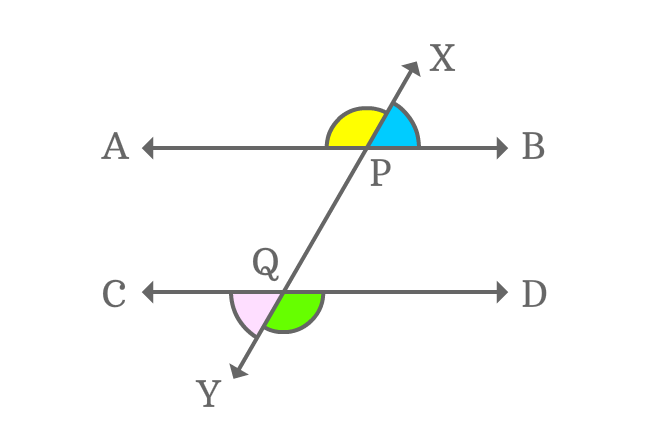# Exterior angles formed by Parallel lines and their TransversalAngles are formed externally when two or more parallel lines are intersected by their transversal and the outside angles are called exterior angles of parallel lines and their transversal.

Observe the picture which expresses an intersection of two parallel lines and their transversal. The intersection of them formed four angles externally. Therefore, they are known as exterior angles of the parallel lines and their transversal.

## Exterior anglesFour exterior angles are formed geometrically when two parallel lines are cut by their transversal.

$1. \,\,\,\,\,\,$ $\angle APX$ is first exterior angle

$2. \,\,\,\,\,\,$ $\angle XPB$ is second exterior angle

$3. \,\,\,\,\,\,$ $\angle DQY$ is third exterior angle

$4. \,\,\,\,\,\,$ $\angle YQC$ is fourth exterior angle

The four angles are formed externally when two parallel lines are cut by a straight line. So, they are called as exterior angles.

### PropertyThe exterior angles which appear in opposite side alternatively are equal geometrically when a pair of parallel lines cut by a transversal.

$1. \,\,\,\,\,\,$ $\angle APX = \angle DQY$

$2. \,\,\,\,\,\,$ $\angle XPB = \angle YQC$

The property of exterior angles is often useful to us while studying the geometrical mathematics and its related subjects.

Latest Math Topics
Jun 26, 2023
Jun 23, 2023

###### Math Questions

The math problems with solutions to learn how to solve a problem.

Learn solutions

Practice now

###### Math Videos

The math videos tutorials with visual graphics to learn every concept.

Watch now

###### Subscribe us

Get the latest math updates from the Math Doubts by subscribing us.